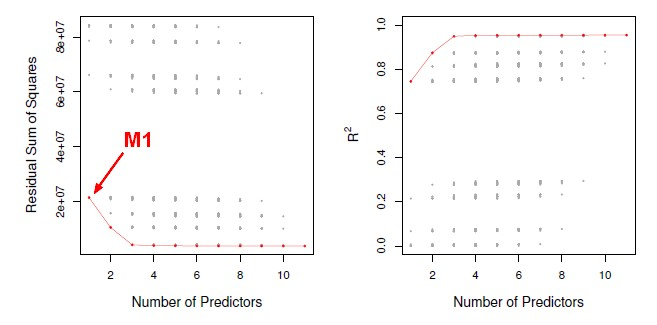# Statistics - Mallow's Cp

## Formula

$$\begin{array}{rrl} C_p & = & \frac{1}{n}(\href{RSS}{RSS} +2d \hat{\sigma}^2) \end{array}$$ where:

• d is the total # of parameters used
• $\hat{\sigma}^2$ is the variance estimate of the of the error associated with each response measurement (ie each error epsilon in the linear model)

## Restrictions

Cp is restricted to cases where n is bigger than p. If p is bigger than n, there is a problem because the full model (ie with all p predictors) is not defined and the error will be zero. Even if p is close to n, there will be a problem because the estimate of sigma squared might be far too low.

Recommended PagesData Mining - (Test|Expected|Generalization) Error

Test error is the prediction error that we incur on new data. The test error is actually how well we'll do on future data the model hasn't seen. The test error is the average error that results from using...Machine Learning - (Overfitting|Overtraining|Robust|Generalization) (Underfitting)

A learning algorithm is said to overfit if it is: more accurate in fitting known data (ie training data) (hindsight) but less accurate in predicting new data (ie test data) (foresight) Ie the model...R - Feature Selection - Indirect Model Selection

In a feature selection process, once you have generated all possible models, you have to select the best one. This article talks the indirect methods. We will select the models using CP but as...R - Feature selection - Model Generation (Best Subset and Stepwise)

This article talks the first step of feature selection in R that is the models generation. Once the models are generated, you can select the best model with one of this approach: Best subset regressiosigreedy...A big R squared indicates a model that really fits the data well. But unfortunately, you can't compare models of different sizes by just taking the one with the biggest R squared because you can't compare...Statistics - Akaike information criterion (AIC)

AIC stands for Akaike Information Criterion. Akaike is the name of the guy who came up with this idea. AIC is a quantity that we can calculate for many different model types, not just linear models,...Statistics - Bayesian Information Criterion (BIC)

BIC is like AIC and Mallow's Cp, but it comes from a Bayesian argument. The formulas are very similar. The formula calculate the residual sum of squares and then add anadjustment term which is...Statistics - Model Selection

Model selection is the task of selecting a statistical model from a set of candidate models through the use of criteria's Dimension reduction procedures generates and returns a sequence of possible...